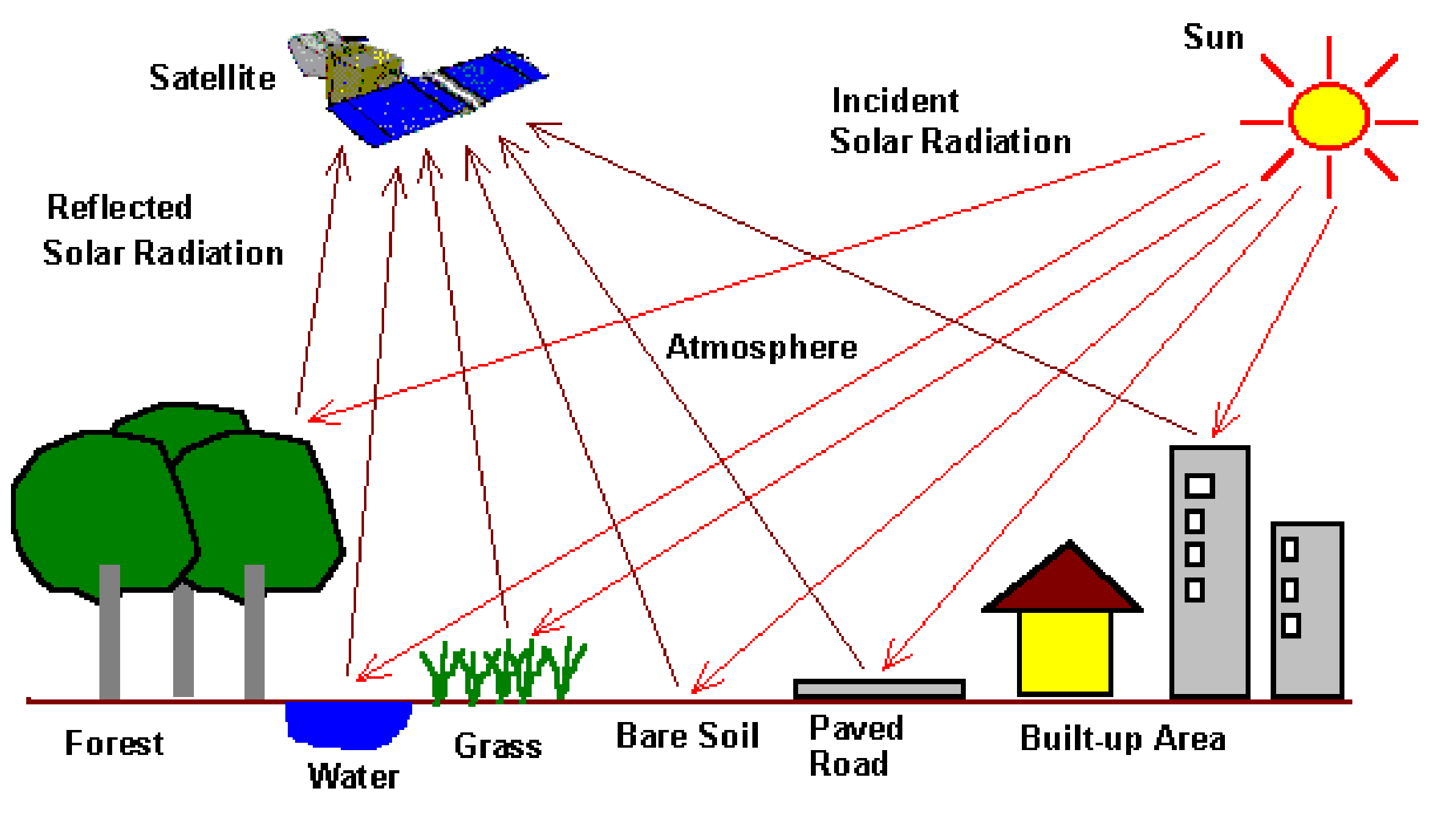Different materials reflect and absorb wavelengths differently. Remote sensing utilizes this phenomena to capture a picture of the Earth’s surface by detecting how various forms of ground cover reflect solar radiation.

In the diagram below for example, the built-up area, bare soil, and forest will absorb the sun’s radiation differently and reflect it in a way that makes them unique to the sensors on the satellite. We can then view these differences in the spectral bands of satellite imagery.Raw satellite imagery, however, is not necessarily useful when performing various analyses. To get useful inputs about land cover in an area, we must transform the imagery. One way to do this is to classify the imagery into categories that we are interested in.

This tutorial introduces using rasters and classifying imagery in R. It is based on a similar tutorial from UC Davis. We will cover:

For this tutorial, we use Landsat 8 imagery from Calgary, which can be found here. However, the process can be repeated with any Landsat 8 imagery downloaded from either Earth Explorer or other sites.

``````library(raster)
library(tidyverse)
library(sf)
library(rpart)
library(rpart.plot)
library(rasterVis)
library(mapedit)
library(mapview)
library(caret)
library(forcats)``````

Next, we read in the different bands that comprise the satellite imagery. Each band refers to a different spectrum:

Band Description
Band 1 Coastal aersol
Band 2 Blue
Band 3 Green
Band 4 Red
Band 5 Near Infrared (NIR)
Band 6 Shortwave Infrared (SWIR) 1
Band 7 Shortwave Infrared (SWIR) 2
Band 8 Panchromatic
Band 9 Cirrus
Band 10 Thermal Infrared (TIRS) 1
Band 11 Thermal Infrared (TIRS) 2
``````band1 <- raster("./data/band1.tif")
band2 <- raster("./data/band2.tif")
band3 <- raster("./data/band3.tif")
band4 <- raster("./data/band4.tif")
band5 <- raster("./data/band5.tif")
band6 <- raster("./data/band6.tif")
band7 <- raster("./data/band7.tif")
band8 <- raster("./data/band8.tif")
band9 <- raster("./data/band9.tif")
band10 <- raster("./data/band10.tif")
band11 <- raster("./data/band11.tif")``````

To perform any analysis, however, we need to combine the individual bands into one multi-band raster. The `stack` function in the `raster` package will create one raster from the designated layers, similar to the composite bands function in ArcGIS.

Note that band 8, the Panchromatic image, is calculated at a different resolution (15 meters rather than 30 meters) than the other bands.

``res(band8)``
``##  15 15``

If we try to `stack` this band with the others as is, it will return an error. We `aggregate` the cell size to 30 meters prior to stacking the rasters into one image.

``````band8 <- aggregate(band8, fact = 2)

image <- stack(band1, band2, band3, band4, band5, band6, band7,
band8, band9, band10, band11)``````

## Exploring the imagery

There are several properties of the raster we can access in R that would normally be found in the properties window in ArcGIS. Below, we look at the number of layers (or bands) the raster has, the coordinate system the imagery is projected in, and the resolution (or grid cell size) of the raster.

``nlayers(image)``
``##  11``
``crs(image)``
``````## CRS arguments:
##  +proj=utm +zone=12 +datum=WGS84 +units=m +no_defs +ellps=WGS84 +towgs84=0,0,0``````
``res(image)``
``##  30 30``

Now that we know a little more about the imagery we are using, let’s plot it. Since `image` is a multi-band raster, we use the `plotRGB` function from the `raster` package, which allows us to specify what bands should be visualized.

There are two main composites that are normally used in remote sensing: the true color composite and the false color composite. As the name suggests, the true color composite plots the imagery as it would appear to the human eye. It uses the red band (4) for red, the green band (3) for green, and the blue band (2) for blue.

``````par(col.axis="white",col.lab="white",tck=0)
plotRGB(image, r = 4, g = 3, b = 2, axes = TRUE,
stretch = "lin", main = "True Color Composite")
box(col="white")``````